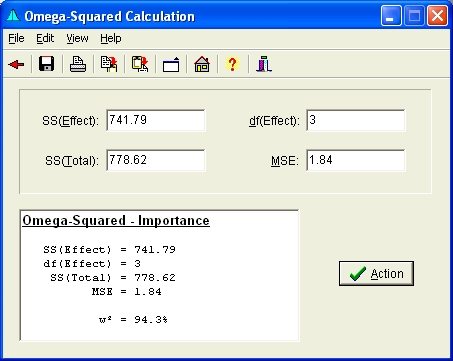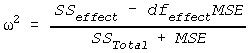# Omega-Squared

The Omega-Squared Form performs calculation of, the importance of a Fixed Factor in an Analysis of Variance.## CalculationWhere:

• SS(Effect) is the Sum of Squares of the effect of interest,
• df(Effect) is the degrees of freedom of the effect of interest,
• SS(Total) is the Total Sum of Squares in the ANOVA, and
• MSE is the Mean-Square Error term.